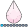NKormanik
Barite | Level 11

## Merge Several Datasets Having The Same Variable Names

I want to merge 9 files.

Each of the files has the same 3 variables:

X, Y, Percent  (out datasets from 9 Proc Freq runs)

Two issues come up:

-- Do I have to rename Y in each of the files beforehand?  (Doesn't SAS automatically do that?)

-- I don't want to keep the Percent column in any of the files. How to most easily dump that one?

``````data merged;
merge one
merge two
merge three;
.
merge nine
by X;
run
``````

Any help greatly appreciated.

Nicholas Kormanik

1 ACCEPTED SOLUTION

Accepted SolutionsKsharp
Super User

## Re: Merge Several Datasets Having The Same Variable Names

You have to rename Y since SAS would not do it for you, Keep the variables you need.

``````data merged;
merge
one(keep=x y rename=(y=y1))
two(keep=x y rename=(y=y2)
three(keep=x y rename=(y=y3))
....
nine(keep=x y rename=(y=y9));
by X;
run;``````
2 REPLIES 2Ksharp
Super User

## Re: Merge Several Datasets Having The Same Variable Names

You have to rename Y since SAS would not do it for you, Keep the variables you need.

``````data merged;
merge
one(keep=x y rename=(y=y1))
two(keep=x y rename=(y=y2)
three(keep=x y rename=(y=y3))
....
nine(keep=x y rename=(y=y9));
by X;
run;``````NKormanik
Barite | Level 11

## Re: Merge Several Datasets Having The Same Variable Names

Thank you, Xia Keshan!  Beautifully done.

By the way, really good to see you back here.  What a wonderful asset to this community.

Nicholas

Discussion stats
• 2 replies
• 913 views
• 1 like
• 2 in conversation